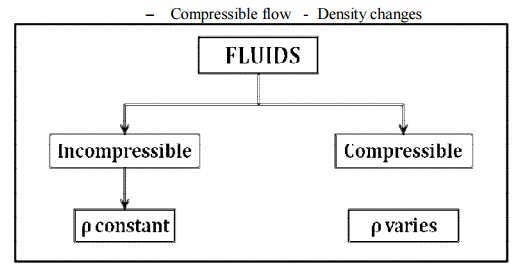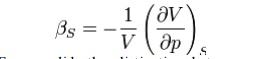Home | | Gas Dynamics and Jet Propulsion | Compressible Flows

# Compressible Flows

In general, liquids are incompressible; water being an excellent example. In contrast compressible fluids do undergo density changes.

Compressible Flows

–  Compressible flow  - Density changesWe know that fluids, such as gas, are classified as Incompressible and Compressible fluids. Incompressible fluids do not undergo significant changes in density as they flow. In general, liquids are incompressible; water being an excellent example. In contrast compressible fluids do undergo density changes. Gases are generally compressible; air being the most common compressible fluid we can find. Compressibility of gases leads to many interesting  features  such  as  shocks, which are absent for incompressible fluids. Gas dynamics is the discipline that studies the flow of compressible fluids and forms an important branch of Fluid Mechanics.

Compressible vs. Incompressible Flow

o   A flow is classified as incompressible if the density remains nearly constant.

o   Liquid flows are typically incompressible.

o   Gas flows are often compressible, especially for high speeds.

o   Mach number, Ma = V/c is a good indicator of whether or not compressibility effects are important.

·        Ma < 0.3 : Incompressible

·        Ma < 1 : Subsonic

·        Ma = 1 : Sonic

·        Ma > 1 : Supersonic

§  Ma >> 1 : Hypersonic

Compressibility:

Measure of the relative volume change with pressureA measure of the relative volume change with pressure for a given process. Consider a small element of fluid of volume v, the pressure exerted on the sides of the element is p. Assume the pressure is now increased by an infinitesimal amount dp. The volume of the element will change by a corresponding amount dv , here the volume decrease so dv is a negative quantity. By definition, the compressibility of fluid isThe terms compressibility and incompressibility describe the ability of molecules in a fluid to be compacted or compressed (made more dense) and their ability to bounce back to their original density, in other words, their "springiness." An incompressible fluid cannot be compressed and has relatively constant density throughout. Liquid is an incompressible fluid. A gaseous fluid such as air, on the other hand, can be either compressible or incompressible. Generally,   for   theoretical   and   experimental purposes,  gases are  assumed to  be incompressible when they are moving at low speeds--under approximately 220 miles per hour. The motion of the object traveling through the air at such speed does not affect the density of the air. This assumption has been useful in aerodynamics when studying the behavior of air in relation to airfoils and other objects moving through the air at slower speeds.

In thermodynamics and fluid mechanics, compressibility is a measure of the relative volume change of a fluid or solid as a response to a pressure (or mean stress) change.Where V is volume and p is pressure. The above statement is incomplete, because for any object or system the magnitude of the compressibility depends strongly on whether the process is adiabatic or isothermal. Accordingly we define the isothermal compressibility as:Where the subscript T indicates that the partial differential is to be taken at constant temperature. The adiabatic compressibility as:Where S is entropy. For a solid, the distinction between the two is usually negligible. The inverse of the compressibility is called the bulk modulus, often denoted K(sometimes B).

Compressibility and Incompressibility

The terms compressibility and incompressibility describe the ability of molecules in a fluid to be compacted or compressed (made more dense) and their ability to bounce back to their original density, in other words, their "springiness." An incompressible fluid cannot be compressed and has relatively constant density throughout. Liquid is an incompressible fluid. A gaseous fluid such as air, on the other hand, can be either

compressible or incompressible. Generally,   for   theoretical   and   experimental purposes,  gases are  assumed  to  be incompressible when they are moving at low speeds--under approximately 220 miles per hour. The motion of the object traveling through the air at such speed does not affect the density of the air. This assumption has been useful in aerodynamics when studying the behavior of air in relation to airfoils and other objects moving through the air at slower speeds.

However, when aircraft began traveling faster than 220 miles per hour, assumptions regarding the air through which they flew that were true at slower speeds were no longer valid. At high speeds some of the energy of the quickly moving aircraft goes into compressing the fluid (the air) and changing its density. The air at higher altitudes where these aircraft fly also has lower density than air nearer to the Earth's surface. The airflow is now compressible, and aerodynamic theories have had to reflect this. Aerodynamic theories relating to compressible airflow characteristics and behavior are considerably more complex than theories relating to incompressible airflow. The noted aerodynamicist of the early 20th century, Ludwig Prandtl, contributed the Prandtl-Glaubert rule for subsonic airflow to describe the compressibility effects of air at high speeds. At lower altitudes, air has a higher density and is considered incompressible for theoretical and experimental purposes.Compressibility

·        Compressibility of any substance is the measure of its change in volume under the action of external forces.

·        The normal compressive stress on any fluid element at rest is known as hydrostatic pressure p and arises as a result of innumerable molecular collisions in the entire fluid.

·        The degree of compressibility of a substance is characterized by the bulk modulus of elasticity E defined asWhereand Δpare the changes in the volume and pressure respectively, and is the initial volume. The negative sign (-sign) is included to make E positive, sinceincrease in pressure would decrease the volume i.e for Δp>0, <0) in volume.

Study Material, Lecturing Notes, Assignment, Reference, Wiki description explanation, brief detail
Mechanical : Gas Dynamics and Jet Propulsion : Basic Concepts and Isentropic Flows : Compressible Flows |